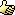# Thread: How do I round a number DOWN?

1. ## How do I round a number DOWN?

does anyone know how to round a number down? (like 17.9 to 17 not 18 etc) I'm in a college lesson and I cant seem to find out how to do it, its probably something really simple that i haven't thought of!Reply With Quote

2. ## Re: How do I round a number DOWN?Originally Posted by Cuffbertt
does anyone know how to round a number down? (like 17.9 to 17 not 18 etc) I'm in a college lesson and I cant seem to find out how to do it, its probably something really simple that i haven't thought of!If its allways going to be rounded down then is this true...

17.9 - 17
18.2 - 18
16 .8 - 16

etc etc

then the number will be just remove the decimal place?

So...

VB Code:
```dim temp() as string
dim inumber as string
Temp = split(iNumber,".")
Msgbox temp(0)```

Try that, I'm not sure if there is a specific function out there for this.Reply With Quote

3. ## Re: How do I round a number DOWN?

VB Code:
```Dim x As String
Dim inum As Long
x = "17.9"
inum = CLng(Split(Trim(x), ".")(0))
iround = Round(CLng(x))
MsgBox inum & " " & iround
If inum < iround Then MsgBox inum```
hehe, jst trying...Reply With Quote

4. ## Re: How do I round a number DOWN?

Thanks!Reply With Quote

5. ## Re: How do I round a number DOWN?

Please don't use string conversions for a simple task like this. Users can set a decimal seperator in the control panel, so you can't assume the period is the decimal seperator.

VB has two functions for rounding down: Int and Fix

For positive numbers, both return the same results, and round the number down.
eg
Fix(12.9) = 12
Int(12.9) = 12
For negative numbers, Int rounds down, and Fix rounds up
eg
Fix(-12.9) = -12
Int(-12.9) = -13Reply With Quote

6. ## Re: How do I round a number DOWN?

To proof my point, I tested pino's code like this:
VB Code:
```Dim temp() As String
Dim inumber As String
Dim dblNum As Double
dblNum = 12.9
inumber = CStr(dblNum)
temp = Split(inumber, ".")
MsgBox temp(0)```

The messagebox shows 12,9
I have the comma as decimal seperator (a standard Dutch setting)Reply With Quote

7. ## Re: How do I round a number DOWN?

assuming you convert the number to string before hand the code would work fine. Also you just use the type of decimal place your program is outputing...

As for your test, just change the . to ,

Its not that complexReply With Quote

8. ## Re: How do I round a number DOWN?

I did convert the number to a string, using the CStr function.
I prefer my code to work with all settings, so using your code, probably some api's are needed to find out what character is set as the decimal seperator in the system.

Also your code is much slower, because it needs string conversions.Reply With Quote

9. ## Re: How do I round a number DOWN?Originally Posted by Frans C
I did convert the number to a string, using the CStr function.
I prefer my code to work with all settings, so using your code, probably some api's are needed to find out what character is set as the decimal seperator in the system.

Also your code is much slower, because it needs string conversions.
I understand your points but in this situation is the speed diferance (minimal differance) going to have a big effect of the program?

I doubt it..

:-/Reply With Quote

####Posting Permissions

• You may not post new threads
• You may not post replies
• You may not post attachments
• You may not edit your posts
•